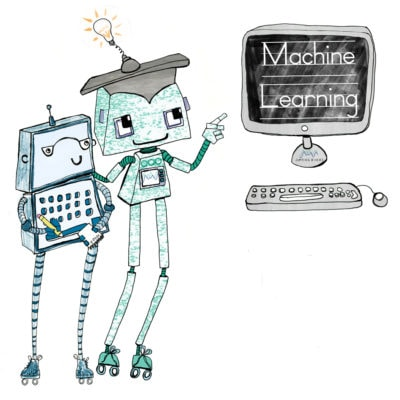GitHub

# Machine Learning with PythonCourse Level: Intermediate

Python (along with R) has become the dominant language in machine learning and data science. This two-day intensive course will equip you with the knowledge and tools to undertake a variety of tasks in a standard machine learning analytics pipeline. We stress the importance of data preparation, both in terms of data standardisation and feature selection, before tackling model building.

We run a separate course on using Tensorflow with Python.

## Book: Machine Learning with Python

 Start Date: Price: Venue Details: Time: Duration:

## Course Details

• Course Outline
• Learning Outcomes
• Materials
• Prior Knowledge
• Introduction to Machine Learning: An introduction to machine learning and the associated packages in Python, such as Numpy, Scipy, and SciKit-Learn.
• Data Preprocessing: Learn the why and how about preprocessing your data with scaling transformations and one hot encoding. We cover typical standardisation and normalisation procedures.
• Introduction to Modelling: Introductory modelling techniques such as linear regression and how we move from a statistical model to a machine learning model.
• Model Assessment: Quantify the effectiveness of your models using training, validation and test sets plus techniques such as cross-validation. We discuss the different metrics that can be used to judge a model and which are appropriate.
• Regularisation: Techniques to avoid overfitting and to perform feature selection, such as lasso, ridge and elastic net regression.
• Clustering: An unsupervised learning technique for uncovering patterns and structure within data.
• Advanced Techniques: Some more advanced model fitting using algorithms such as gradient boosted trees and support vector machines.

Session 1:

By the end of session 1, participants will…

• understand the basic premise of machine learning and statistical models.
• have knowledge of using sklearn and linear regression, including:
• model fitting
• interpretation and visualisation of outputs
• scaling and preprocessing data

Session 2:

By the end of session 2, participants will…

• be able to use cross validation with sklearn to assess their model.
• understand what penalised regression is, why it is used, and how it can be implemented with sklearn.

Session 3:

By the end of session 3, participants will…

• know what classification models are and have experience with:
• logistic regression
• linear discriminant analysis and quadratic discriminant analysis
• K-nearest neighbour models
• decision trees
• understand more advanced models including random forest and SVM models, and how to implement them for classification problems.

Session 4:

By the end of session 4, participants will…

• know what clustering is.
• be able to differentiate between the different distance metrics.
• understand hierarchical clustering and K-means clustering, and be able to implement their own models.

This course does not include:

•••••It is expected that participants are comfortable using the Python programming language and common data structures. Some exposure to common statistical terms would be an advantage, but not essential. Attendance of the Introduction to Python course or equivalent experience should be sufficient.

## Attendee Feedback

• “The course was well designed and structured, for us students who know little ML. It introduced me to new tools that I will definitely make use of in my research. Jack was also a great tutor, very knowledgeable and patient with our questions.”
• “The balance between theory and the practicals to apply what we had just learned was great.”
• “Jack was great in answering all our theoretical questions so that we could get a better grasp of the underlying concepts and intuition.”